## Thursday, July 25, 2019

### Shrinkage in hierarchical models: random effects in lmer() with and without correlation

The goal of this post is to illustrate shrinkage of parameter estimates in hierarchical (aka multi-level) models, specifically when using lmer() with and without estimated correlation of parameters. The examples will show how estimates can differ when including correlation of parameters because of shrinkage toward the estimated correlation.

# Background

## Data structure for these examples

“It all begins with the data… ” I will create multiple panels of $\langle x , y \rangle$ data values, with $x$ and $y$ being continuous metric variables.
• For instance, each panel could be data from a student in a classroom, with each datum being performance on a standardized math exam, with $x$ being time and $y$ being performance. In this scenario, each student takes a novel variant of the test repeatedly across time. The times do not need to be the same for every student, and the number of tests do not need to be the same for every student. We are interested characterizing the performance trend of each panel (i.e., each student) and the overall trend across panels (i.e., for the class as a whole).
• As another example, each panel could be data from a distinct class within a school, with each datum being a particular student's exam performance (on the $y$ axis) and family income (on the $x$ axis). Again we are interested characterizing the trend of each panel (i.e., the relation of exam performance to family income within each classroom) and the overall trend across panels (i.e., the typical relationship of the variables across classrooms).
To illustrate robust shrinkage of panel estimates, each panel will have relatively few data points, and there will be relatively lots of panels. Graphs of the data will appear in analysis results, later.

Here (below) is the structure of the data. Notice there is an X variable, a Y variable, and a Panel variable. The panel variable is actually a nominal (categorical) value, even though it appears as a numerical index.
str( myData )

## 'data.frame':    208 obs. of  3 variables:
##  $X : num 0.4158 0.3795 0.0746 0.0588 0.4503 ... ##$ Y    : num  -0.864 -0.579 0.227 -1.604 -0.895 ...
##  \$ Panel: Factor w/ 35 levels "1","2","3","4",..: 1 1 1 1 1 1 2 2 2 3 ...


## Analysis models

For simplicity, each panel will be fit with a linear trend. The hierarchical (a.k.a. multi-level) models will also estimate the typical linear trend across panels.

Parameters for panels are subject to shrinkage in hierarchical models because the panel's linear trend is trying to conform simultaneously to (a) the data in its panel and (b) the typical trend across all panels. When there are lots of panels informing the typical trend, and only a small amount of data within a panel, then the panel estimates are strongly influenced by the typical trend across panels. This makes good sense: If you don't know much about a particular panel, your best estimate should take into account what's typical across many other similar panels.

For more background about shrinkage in hierarchical models, there are lots on online sources you can search, and you can see some of my previous writings on the topic:
I will first fit a line independently to each panel, without hierarchical structure. This analysis will show the estimated intercept and slope in each panel when there is no shrinkage.

I will then fit a hierarchical model that estimates a typical intercept and typical slope across panels, but does not estimate the correlation of the intercepts and slopes across panels. This model produces some shrinkage across panel estimates, but does not shrink the estimates toward a shared correlation across panels.

Finally, I will fit a hiearchical model that also estimates the correlation of intercepts and slopes across panels. This model shrinks the panel estimates so they also conform more strongly with the estimated correlation across panels.

For the non-hierarchical analysis, I will use lm() from the base stats package of R. For the hierarchical analyses, I will use lmer() from the lme4 package in R.

# Independent line for every panel

For this analysis, each individual panel is fit with its own line, separately from all other panels, using lm() on each panel. There is no hierarchical structure and no overall line estimated.

To make this analysis most analogous to subsequent analyses with lmer() the analyses should require all panels to have the same noise variance. But this is not done here, and actually the MLE coefficients are not affected in this case.

In principle, the analysis in this section would be like using lmer() with the formula y ~ 0 + (1+X||Panel), which specifies fitting lines within panels with no estimation of correlation across panels and no global parameters. But lmer() throws an error if that specification is attempted.
Here (below) are scatter plots of the data with the lm() fitted regression lines: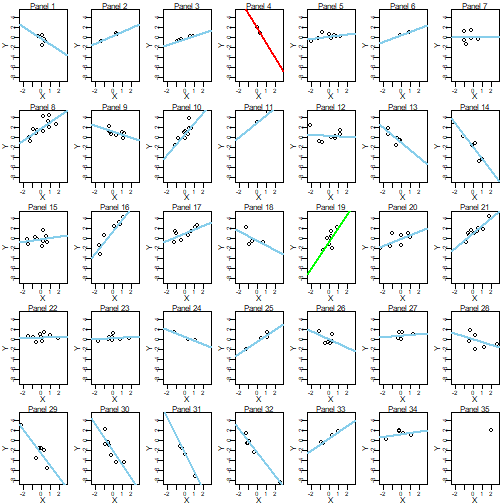Notice above:
• Two-point panels such as Panel 4 and Panel 11 have lines going exactly through the two points. This will not be the case in hierarchical models.
• The one-point Panel 35 has no regression line because it's undefined. This will not be the case in hierarchical models.
• Panels 4 and 19 are color-highlighted for easy comparison with subsequent analyses.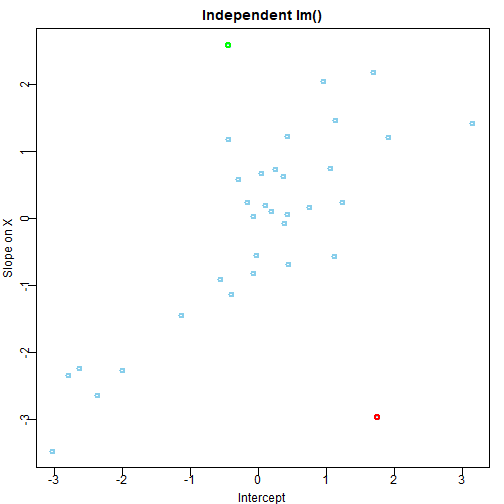Notice above:
• There is correlation of intercepts and slopes across panels (r=0.65), reflecting only how the data were generated, not any estimation of correlation in the model.
• There is a lot of variation in intercepts and slopes across panels relative to hierarchical (multi-level) models below. There will be less variation in hierarchical models, hence the term shrinkage.
• Panels 4 and 19 are color-highlighted for easy comparison across analyses.

# Random intercepts and slopes, but no estimated correlation

I'll use lmer() with the formula, Y ~ 1 + X + ( 1 + X || Panel ), which is equivalent to Y ~ 1 + X + ( (1|Panel) + (0+X|Panel) ). lmer() assumes we want to estimate correlations of parameters across panels unless we tell it not to by using a double vertical bar or by explicitly coding the separate effects.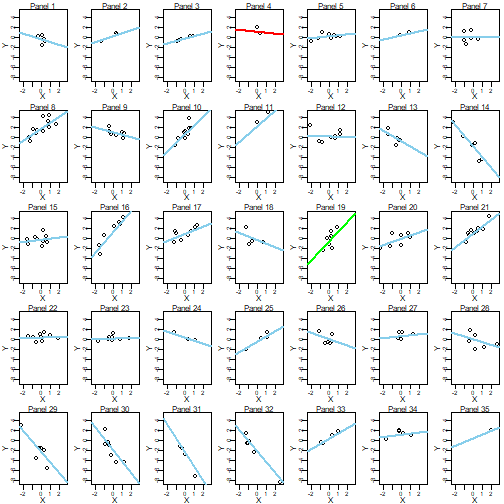Notice above:
• Two-point panels such as Panel 4 and Panel 11 have lines not going exactly through the two points. This is because the line is trying to conform simultaneously to the data in the panel and what's typical across panels, as estimated by this particular hierarchical model.
• The one-point Panel 35 has a regression line despite having only a single point. This is because the line is generated by what's typical across panels, influenced a bit by the single data point in the panel.
• Panels 4 and 19 are color-highlighted for easy comparison with across analyses.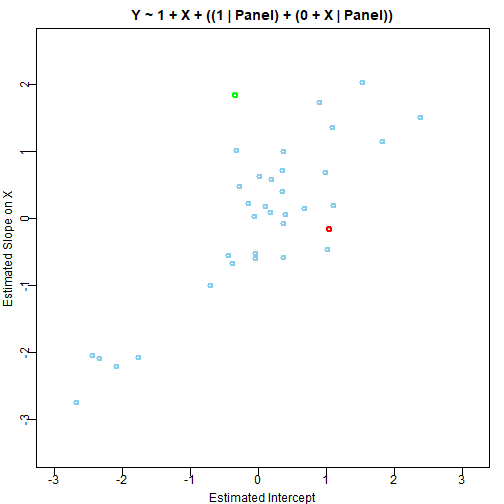Notice above:
• There is correlation of intercepts and slopes across panels (r=0.814), but this reflects only how the data were generated and the separate shrinkage of intercepts and slopes, without any shrinkage from estimation of correlation.
• There is less variation in intercepts and slopes across panels relative to the previous, non-hierarchical analysis, hence the term shrinkage. Specifically, the range of slopes across panels in the non-hierarchical model was -3.48, 2.59 but the range of slopes in this hierarchical model is -2.74, 2.03.
• Panels 4 and 19 are color-highlighted for easy comparison across analyses.

# Random intercepts and slopes, with estimated correlation

Here I use lmer() with forumla Y ~ 1 + X + ( 1 + X | Panel ). Notice the single vertical bar before Panel, so lmer() estimates the correlation of parameters across panels by default.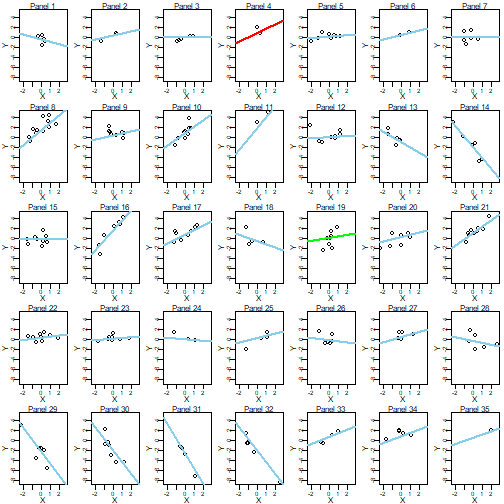Notice above:
There is even more shrinkage than in the previous model, because now the lines in each panel are also “trying” to conform to the typical correlation of intercept and slope across panels. Notice in particular the color-coded lines in panels 4 and 19.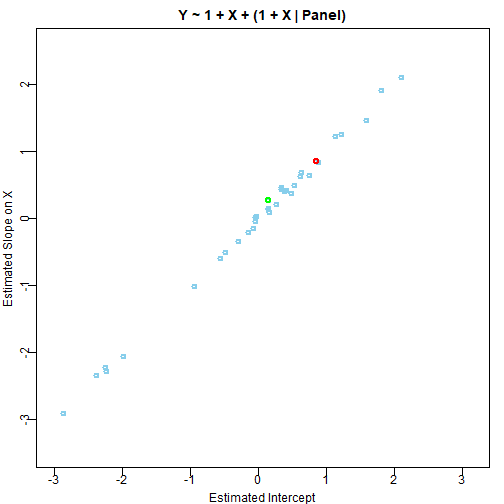Notice above:
There is a strong correlation between the estimated slopes and intercepts (r=0.998). Here the correlation is estimated and there is shrinkage of estimates toward that correlation, and the correlation is stronger than the previous model because the estimates are shrunken toward that correlation.

# What about the higher-level, "fixed" effects?

The higher-level intercept and slope are at the means of the panel intercepts and panel slopes, and those overall means are essentially the same across these analyses.

# Conclusion

Hopefully these examples have helped to illustrate shrinkage in hierarchical models, and specifically the extra shrinkage introduced by estimating correlation across parameters.

1.Quite interesting illustration, thanks for this post.

I've something still unclear, when you distinguish "correlations due to the data generation" and "correlations between estimates". I'm not sure I understand the origin of both correlations.
In particular, I didn't saw in the data generation process if the slope and intercept were generated for each panel using independant or correlated random effects.
In the first case, I do not understand what the correlation obtained using correlated random effects mean exactly...
Could you please give a few explainations?
1.